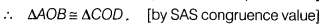# Prove that (i) AC and BD are diameters. (ii) ABCD is a rectangle

AC and BD are chords of a circle which bisect each other at O. Prove that
(i) AC and BD are diameters.
(ii) ABCD is a rectangle.

Given AC and BD are chords of a circle which bisect each other at O.
(i)AC and BD are diameters.
(ii)ABCD is a rectangle
Proof
(i) In ∆AOS and ∆COD, we have
OA=OC [∵ O is the mid-point of AC]
∠AOB=∠COD
OB = OD [∵ O is the mid-point of BD]⇒ CD = AB [by CPCT]
∴ CD = AB …(i)
Similarly, in ∆AOD and ∆COS, we have